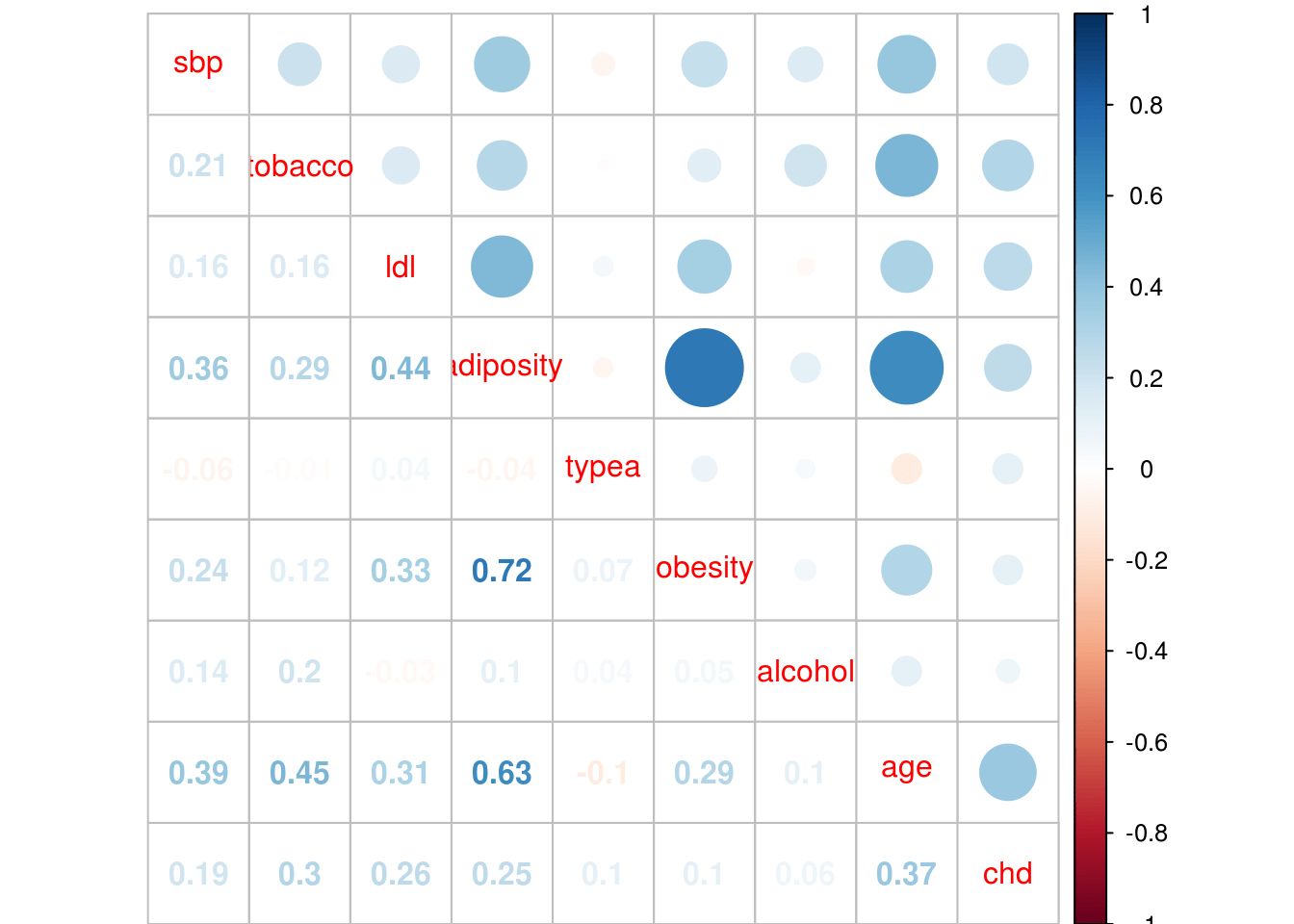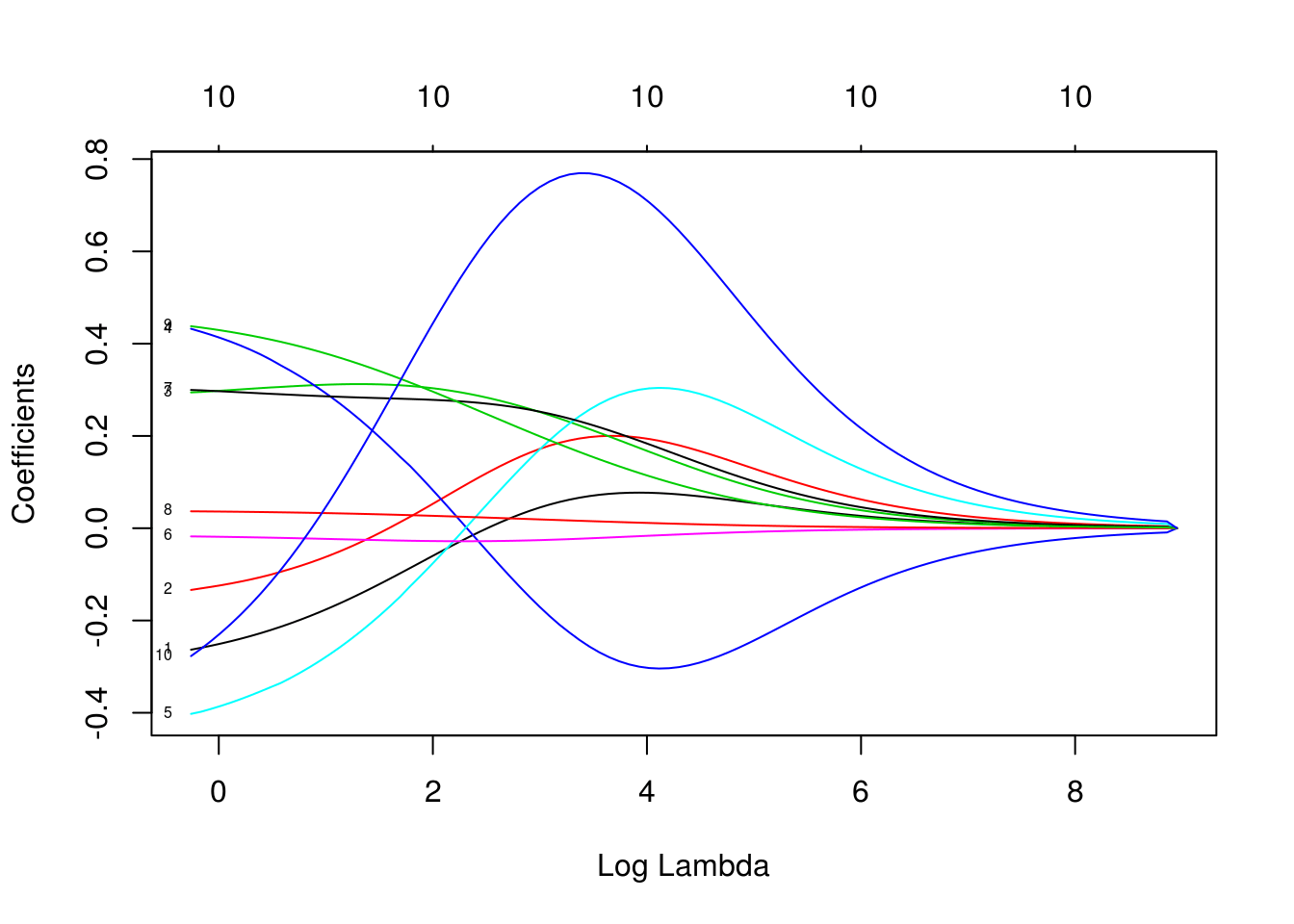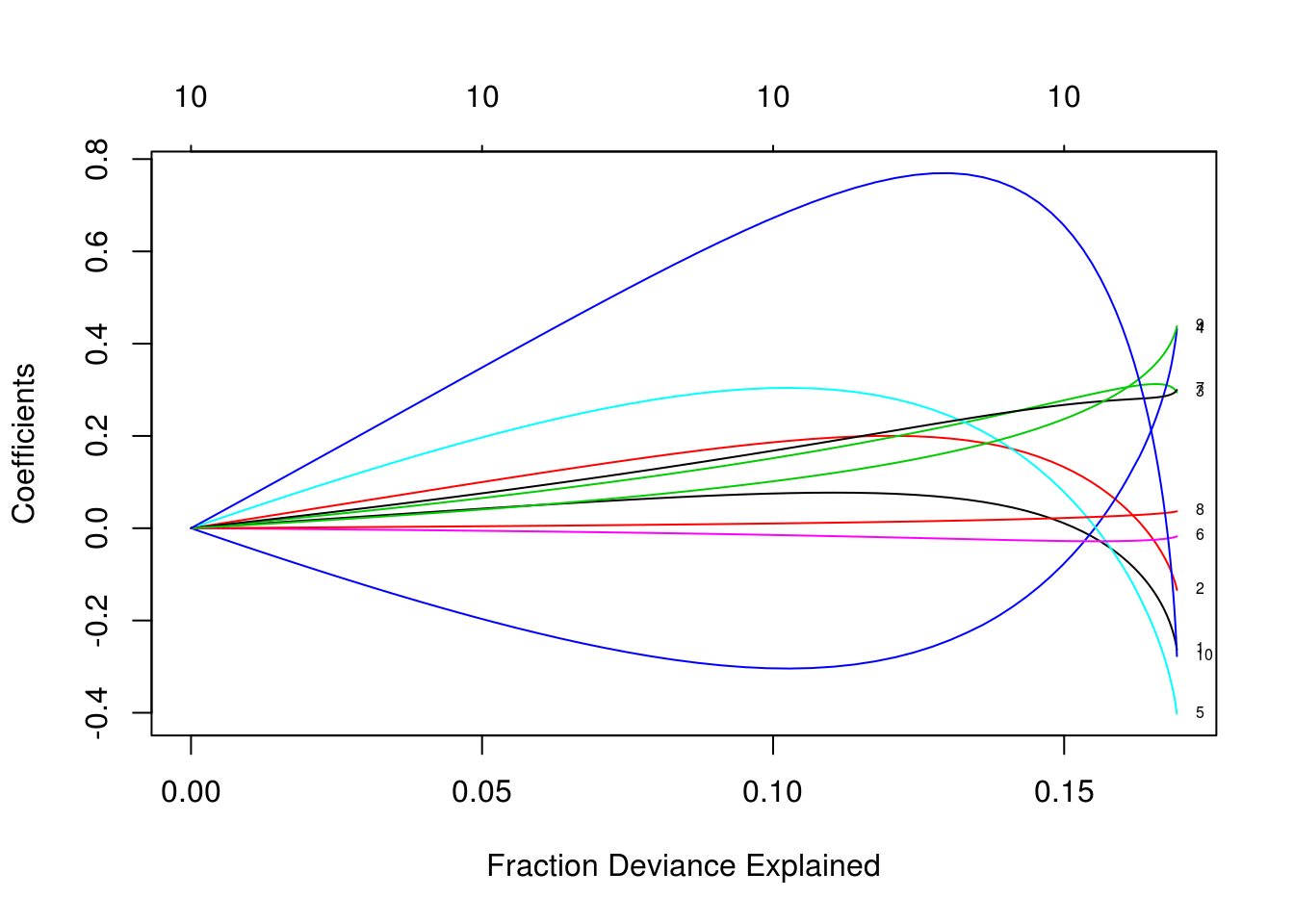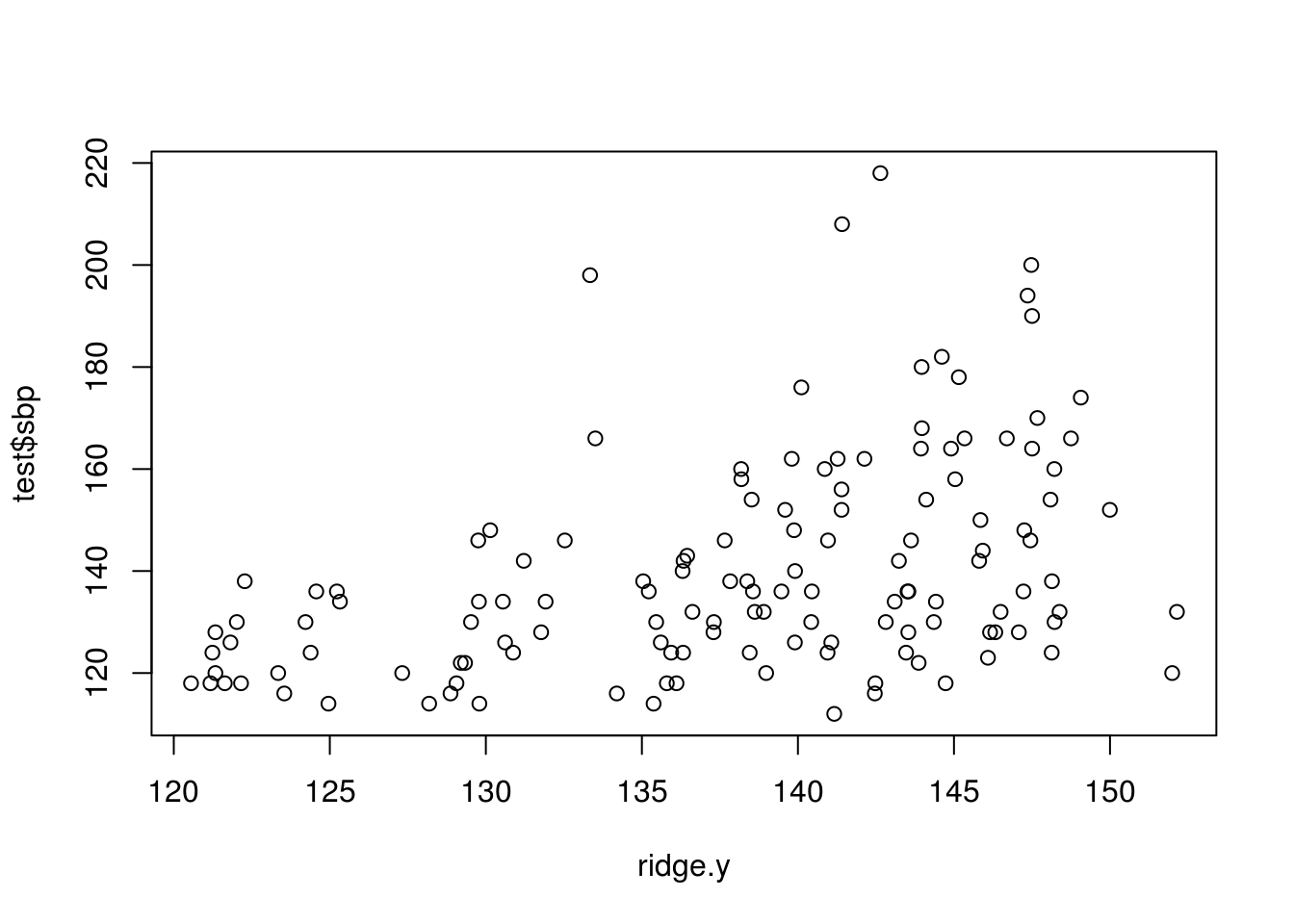# Ridge Regression in R

In this post, we will conduct an analysis using ridge regression. Ridge regression is a type of regularized regression. By applying a shrinkage penalty, we are able to reduce the coefficients of many variables almost to zero whilestill retaining them in the model. This allows us to develop models that have many more variables in them compared to models using the best subset or stepwise regression.

In the example used in this post, we will use the “SAheart” dataset from the “ElemStatLearn” package. We want to predict systolic blood pressure (sbp) using all of the other variables available as predictors. Below is some initial code that we need to begin.

``````library(ElemStatLearn);library(car);library(corrplot)
library(leaps);library(glmnet);library(caret)``````
```data(SAheart)
`str(SAheart)````
``````## 'data.frame':    462 obs. of  10 variables:
##  \$ sbp      : int  160 144 118 170 134 132 142 114 114 132 ...
##  \$ tobacco  : num  12 0.01 0.08 7.5 13.6 6.2 4.05 4.08 0 0 ...
##  \$ ldl      : num  5.73 4.41 3.48 6.41 3.5 6.47 3.38 4.59 3.83 5.8 ...
##  \$ adiposity: num  23.1 28.6 32.3 38 27.8 ...
##  \$ famhist  : Factor w/ 2 levels "Absent","Present": 2 1 2 2 2 2 1 2 2 2 ...
##  \$ typea    : int  49 55 52 51 60 62 59 62 49 69 ...
##  \$ obesity  : num  25.3 28.9 29.1 32 26 ...
##  \$ alcohol  : num  97.2 2.06 3.81 24.26 57.34 ...
##  \$ age      : int  52 63 46 58 49 45 38 58 29 53 ...
##  \$ chd      : int  1 1 0 1 1 0 0 1 0 1 ...``````

A look at the object using the “str” function indicates that one variable “famhist” is a factor variable. The “glmnet” function that does the ridge regression analysis cannot handle factors so we need to convert this to a dummy variable. However, there are two things we need to do before this. First, we need to check the correlations to make sure there are no major issues with multicollinearity Second, we need to create our training and testing data sets. Below is the code for the correlation plot.

``````p.cor<-cor(SAheart[,-5])
corrplot.mixed(p.cor)``````First we created a variable called “p.cor” the -5 in brackets means we removed the 5th column from the “SAheart” data set which is the factor variable “Famhist”. The correlation plot indicates that there is one strong relationship between adiposity and obesity. However, one common cut-off for collinearity is 0.8 and this value is 0.72 which is not a problem.

We will now create are training and testing sets and convert “famhist” to a dummy variable.

``````ind<-sample(2,nrow(SAheart),replace=T,prob = c(0.7,0.3))
train<-SAheart[ind==1,]
test<-SAheart[ind==2,]
train\$famhist<-model.matrix( ~ famhist - 1, data=train ) #convert to dummy variable
test\$famhist<-model.matrix( ~ famhist - 1, data=test )``````

We are still not done preparing our data yet. “glmnet” cannot use data frames, instead, it can only use matrices. Therefore, we now need to convert our data frames to matrices. We have to create two matrices, one with all of the predictor variables and a second with the outcome variable of blood pressure. Below is the code

``````predictor_variables<-as.matrix(train[,2:10])
blood_pressure<-as.matrix(train\$sbp)``````

We are now ready to create our model. We use the “glmnet” function and insert our two matrices. The family is set to Gaussian because “blood pressure” is a continuous variable. Alpha is set to 0 as this indicates ridge regression. Below is the code

``ridge<-glmnet(predictor_variables,blood_pressure,family = 'gaussian',alpha = 0)``

Now we need to look at the results using the “print” function. This function prints a lot of information as explained below.

•  Df = number of variables including in the model (this is always the same number in a ridge model)
•  %Dev = Percent of deviance explained. The higher the better
• Lambda = The lambda used to attain the %Dev

When you use the “print” function for a ridge model it will print up to 100 different models. Fewer models are possible if the percent of deviance stops improving. 100 is the default stopping point. In the code below we have the “print” function. However, I have only printed the first 5 and last 5 models in order to save space.

``print(ridge)``
``````##
## Call:  glmnet(x = predictor_variables, y = blood_pressure, family = "gaussian",      alpha = 0)
##
##        Df      %Dev    Lambda
##   [1,] 10 7.622e-37 7716.0000
##   [2,] 10 2.135e-03 7030.0000
##   [3,] 10 2.341e-03 6406.0000
##   [4,] 10 2.566e-03 5837.0000
##   [5,] 10 2.812e-03 5318.0000
................................
##  [95,] 10 1.690e-01    1.2290
##  [96,] 10 1.691e-01    1.1190
##  [97,] 10 1.692e-01    1.0200
##  [98,] 10 1.693e-01    0.9293
##  [99,] 10 1.693e-01    0.8468
## [100,] 10 1.694e-01    0.7716``````

The results from the “print” function are useful in setting the lambda for the “test” dataset. Based on the results we can set the lambda at 0.83 because this explains the highest amount of deviance at .20.

The plot below shows us lambda on the x-axis and the coefficients of the predictor variables on the y-axis. The numbers refer to the actual coefficient of a particular variable. Inside the plot, each number corresponds to a variable going from left to right in a data-frame/matrix using the “View” function. For example, 1 in the plot refers to “tobacco” 2 refers to “ldl” etc. Across the top of the plot is the number of variables used in the model. Remember this number never changes when doing ridge regression.

``plot(ridge,xvar="lambda",label=T)``As you can see, as lambda increase the coefficient decrease in value. This is how ridge regression works yet no coefficient ever goes to absolute 0.

You can also look at the coefficient values at a specific lambda value. The values are unstandardized but they provide a useful insight when determining final model selection. In the code below the lambda is set to .83 and we use the “coef” function to do this

``````ridge.coef<-coef(ridge,s=.83,exact = T)
ridge.coef``````
``````## 11 x 1 sparse Matrix of class "dgCMatrix"
##                                   1
## (Intercept)            105.69379942
## tobacco                 -0.25990747
## ldl                     -0.13075557
## famhist.famhistAbsent    0.42532887
## famhist.famhistPresent  -0.40000846
## typea                   -0.01799031
## obesity                  0.29899976
## alcohol                  0.03648850
## age                      0.43555450
## chd                     -0.26539180``````

The second plot shows us the deviance explained on the x-axis and the coefficients of the predictor variables on the y-axis. Below is the code

``plot(ridge,xvar='dev',label=T)``The two plots are completely opposite to each other. Increasing lambda cause a decrease in the coefficients while increasing the fraction of deviance explained leads to an increase in the coefficient. You can also see this when we used the “print” function. As lambda became smaller there was an increase in the deviance explained.

We now can begin testing our model on the test data set. We need to convert the test dataset to a matrix and then we will use the predict function while setting our lambda to .83 (remember a lambda of .83 explained the most of the deviance). Lastly, we will plot the results. Below is the code.

``````test.matrix<-as.matrix(test[,2:10])
ridge.y<-predict(ridge,newx = test.matrix,type = 'response',s=.83)
plot(ridge.y,test\$sbp)``````The last thing we need to do is calculated the mean squared error. By it’s self this number is useless. However, it provides a benchmark for comparing the current model with any other models you may develop. Below is the code

``````ridge.resid<-ridge.y-test\$sbp
mean(ridge.resid^2)``````
``##  372.4431``

Knowing this number, we can develop other models using other methods of analysis to try to reduce it as much as possible.